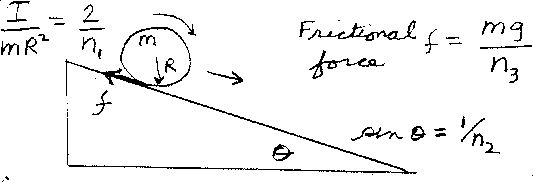Problem D5: An object, of mass m and radius R, rolls without slipping down an incline plane. The incline makes an angle θ with the horizontal, where sin θ = 1/n2. The rotational intertia divided by mR2 of the rolling object is equal to I/(mR2) = 2/n1. What is the frictional force that the incline exerts on the rolling object? If the frictional force equals f = mg/n3, what is n3? Note that for a thin ring, n1=2; for a uniform disk, n1=4; and for a sphere, n1=5. Also, n1, n2, and n3 are unitless.n1 = n2 = Input n3:
If you are currently in my class, you can record your grade by entering your name and student ID number (without the leading zeros) below and clicking on "record grade".
 First Name = Last Name = ID = Problem: